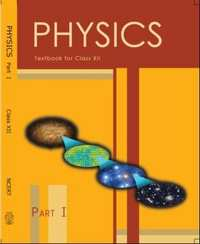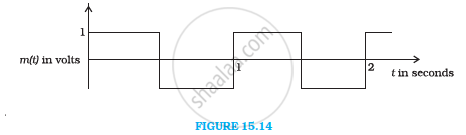# NCERT solutions for Class 12 Physics Textbook chapter 15 - Communication Systems [Latest edition]

#### Chapters## Chapter 15: Communication Systems

Exercise
Exercise [Pages 530 - 531]

### NCERT solutions for Class 12 Physics Textbook Chapter 15 Communication Systems Exercise [Pages 530 - 531]

Exercise | Q 1 | Page 530

Which of the following frequencies will be suitable for beyond-the-horizon communication using sky waves?

(a) 10 kHz

(b) 10 MHz

(c) 1 GHz

(d) 1000 GHz

Exercise | Q 2 | Page 530

Frequencies in the UHF range normally propagate by means of:

(a) Ground waves.

(b) Sky waves.

(c) Surface waves.

(d) Space waves.

Exercise | Q 3 | Page 530

Digital signals

(i) Do not provide a continuous set of values,

(ii) Represent values as discrete steps,

(iii) Can utilize binary system, and

(iv) Can utilize decimal as well as binary systems.

Which of the above statements are true?

(a) (i) and (ii) only

(b) (ii) and (iii) only

(c) (i), (ii) and (iii) but not (iv)

(d) All of (i), (ii), (iii) and (iv).

Exercise | Q 4 | Page 530

Is it necessary for a transmitting antenna to be at the same height as that of the receiving antenna for line-of-sight communication? A TV transmitting antenna is 81m tall. How much service area can it cover if the receiving antenna is at the ground level?

Exercise | Q 5 | Page 530

A carrier wave of peak voltage 12 V is used to transmit a message signal. What should be the peak voltage of the modulating signal in order to have a modulation index of 75%?

Exercise | Q 6 | Page 530

A modulating signal is a square wave, as shownThe carrier wave is given by  c(t) = 2 sin (8pit) "volts"

(i) Sketch the amplitude modulated waveform

(ii) What is the modulation index?

Exercise | Q 7 | Page 531

For an amplitude modulated wave, the maximum amplitude is found to be 10 V while the minimum amplitude is found to be 2 V. Determine the modulation index μ. What would be the value of μ if the minimum amplitude is zero volt?

Exercise | Q 8 | Page 531

Due to economic reasons, only the upper sideband of an AM wave is transmitted, but at the receiving station, there is a facility for generating the carrier. Show that if a device is available which can multiply two signals, then it is possible to recover the modulating signal at the receiver station.

## Chapter 15: Communication Systems

Exercise## NCERT solutions for Class 12 Physics Textbook chapter 15 - Communication Systems

NCERT solutions for Class 12 Physics Textbook chapter 15 (Communication Systems) include all questions with solution and detail explanation. This will clear students doubts about any question and improve application skills while preparing for board exams. The detailed, step-by-step solutions will help you understand the concepts better and clear your confusions, if any. Shaalaa.com has the CBSE Class 12 Physics Textbook solutions in a manner that help students grasp basic concepts better and faster.

Further, we at Shaalaa.com provide such solutions so that students can prepare for written exams. NCERT textbook solutions can be a core help for self-study and acts as a perfect self-help guidance for students.

Concepts covered in Class 12 Physics Textbook chapter 15 Communication Systems are Detection of Amplitude Modulated Wave, Production of Amplitude Modulated Wave, Basic Terminology Used in Electronic Communication Systems, Sinusoidal Waves, Modulation and Its Necessity, Amplitude Modulation (AM), Need for Modulation and Demodulation, Satellite Communication, Propagation of Electromagnetic Waves, Bandwidth of Transmission Medium, Bandwidth of Signals, Elements of a Communication System.

Using NCERT Class 12 solutions Communication Systems exercise by students are an easy way to prepare for the exams, as they involve solutions arranged chapter-wise also page wise. The questions involved in NCERT Solutions are important questions that can be asked in the final exam. Maximum students of CBSE Class 12 prefer NCERT Textbook Solutions to score more in exam.

Get the free view of chapter 15 Communication Systems Class 12 extra questions for Class 12 Physics Textbook and can use Shaalaa.com to keep it handy for your exam preparation